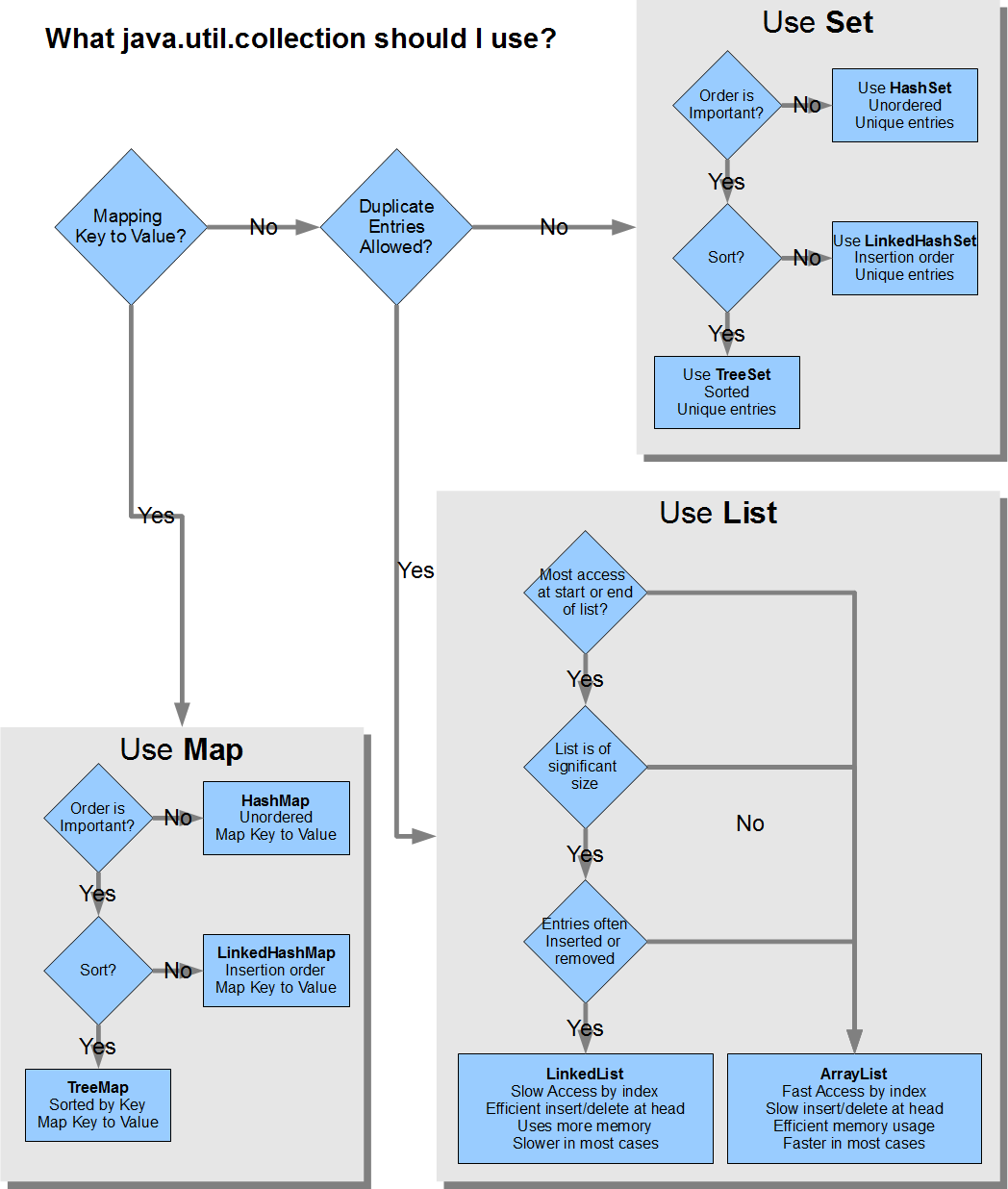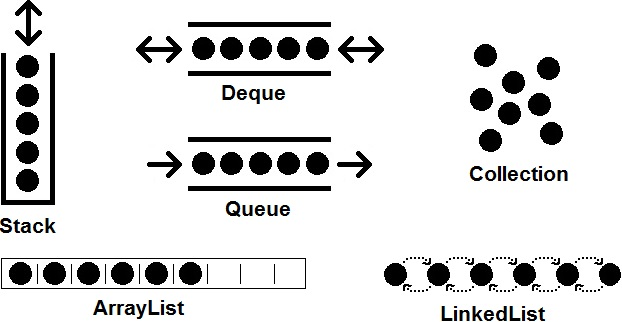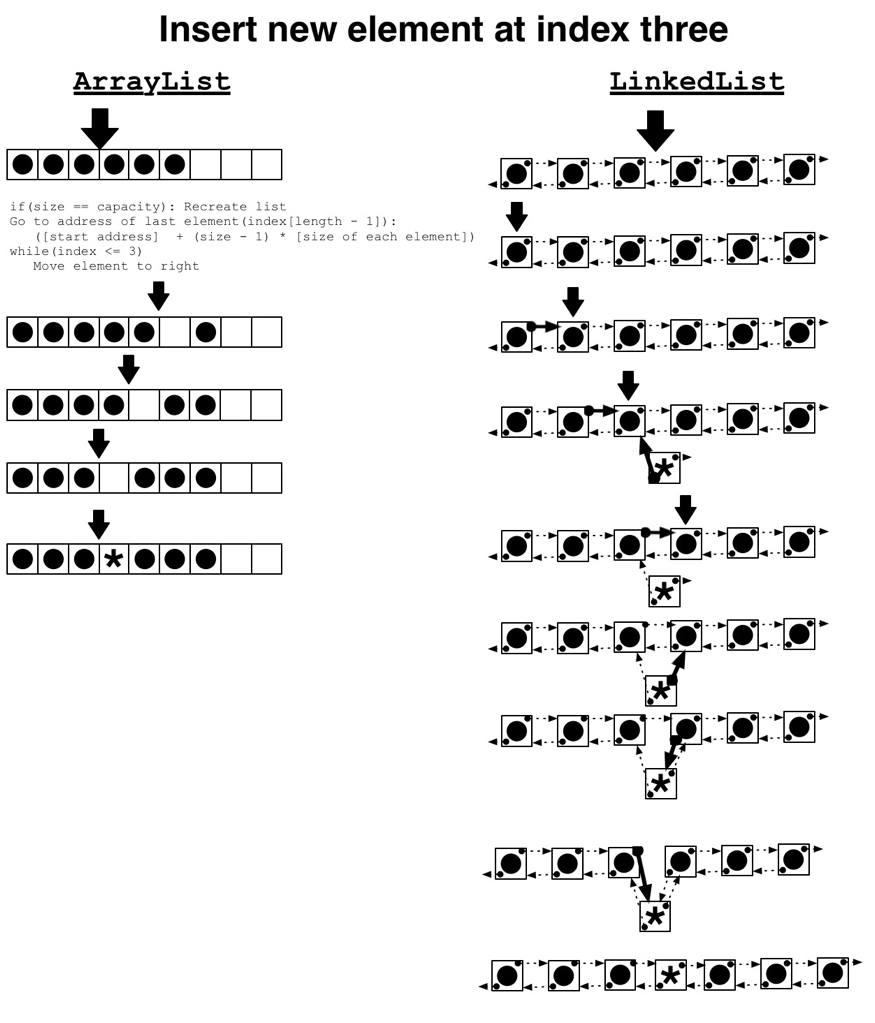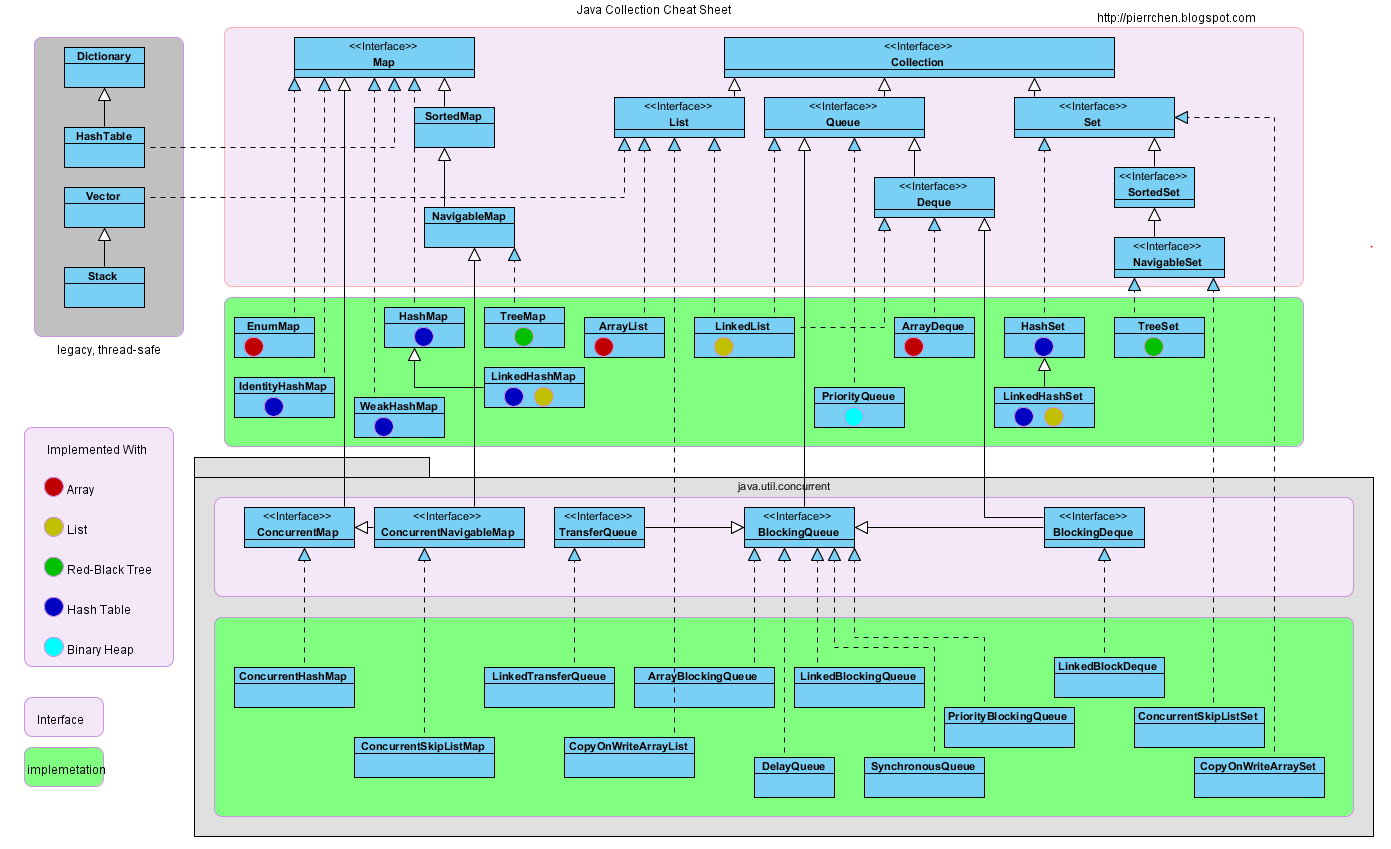Collections (source)

`Collection`: An interface representing an unordered "bag" of items, called "elements". The "next" element is undefined (random).
• `Set`: An interface representing a `Collection` with no duplicates.

• `HashSet`: A `Set` backed by a `Hashtable`. Fastest and smallest memory usage, when ordering is unimportant.
• `LinkedHashSet`: A `HashSet` with the addition of a linked list to associate elements in insertion order. The "next" element is the next-most-recently inserted element.
• `TreeSet`: A `Set` where elements are ordered by a `Comparator` (typically natural ordering). Slowest and largest memory usage, but necessary for comparator-based ordering.
• `EnumSet`: An extremely fast and efficient `Set` customized for a single enum type.

• `List`: An interface representing an `Collection` whose elements are ordered and each have a numeric index representing its position, where zero is the first element, and `(length - 1)` is the last.

• `ArrayList`: A `List` backed by an array, where the array has a length (called "capacity") that is at least as large as the number of elements (the list's "size"). When size exceeds capacity (when the `(capacity + 1)-th` element is added), the array is recreated with a new capacity of `(new length * 1.5)`--this recreation is fast, since it uses `System.arrayCopy()`. Deleting and inserting/adding elements requires all neighboring elements (to the right) be shifted into or out of that space. Accessing any element is fast, as it only requires the calculation `(element-zero-address + desired-index * element-size)` to find it's location. In most situations, an `ArrayList` is preferred over a `LinkedList`.
• `LinkedList`: A `List` backed by a set of objects, each linked to its "previous" and "next" neighbors. A `LinkedList` is also a `Queue` and `Deque`. Accessing elements is done starting at the first or last element, and traversing until the desired index is reached. Insertion and deletion, once the desired index is reached via traversal is a trivial matter of re-mapping only the immediate-neighbor links to point to the new element or bypass the now-deleted element.

• `Map`: An interface representing an `Collection` where each element has an identifying "key"--each element is a key-value pair.
• `HashMap`: A `Map` where keys are unordered, and backed by a `Hashtable`.
• `LinkedhashMap`: Keys are ordered by insertion order.
• `TreeMap`: A `Map` where keys are ordered by a `Comparator` (typically natural ordering).

• `Queue`: An interface that represents a `Collection` where elements are, typically, added to one end, and removed from the other (FIFO: first-in, first-out).
• `Stack`: An interface that represents a `Collection` where elements are, typically, both added (pushed) and removed (popped) from the same end (LIFO: last-in, first-out).
• `Deque`: Short for "double ended queue", usually pronounced "deck". A linked list that is typically only added to and read from either end (not the middle).

Interface: List TS† get add contains next remove(0) iterator.remove Collections Order Complexity (source) ArrayList O(1) O(1) O(n) O(1) O(n) O(n) Array LinkedList O(n) O(1) O(n) O(1) O(1) O(1) Linked List CopyOnWriteArrayList O(1) O(n) O(n) O(1) O(n) O(n) Array HashMap O(1) O(1) O(h/n) Hash Table EnumMap O(1) O(1) O(1) Array LinkedHashMap O(1) O(1) O(1) HT + LL IdentityHashMap O(1) O(1) O(h/n) Array TreeMap O(log(n)) O(log(n)) O(log(n)) RB Tree WeakHashMap O(1) O(1) O(h/n) Hash Table ConcurrentHashMap O(1) O(1) O(h/n) Hash Table ConcurrentSkipListMap O(log n) O(log n) O(1) Skip List HashSet O(1) O(1) O(h/n) O(1) O(1) Hash Table EnumSet O(1) O(1) O(1) O(1) O(1) Bit Vector LinkedHashSet O(1) O(1) O(1) O(1) O(1) HT + LL TreeSet O(log n) O(log n) O(log n) O(log n) O(1) RB Tree ConcurrentSkipListSet O(log n) O(log n) O(1) O(log n) O(n) Skip List CopyOnWriteArraySet O(n) O(n) O(1) O(n) O(1) Array PriorityQueue O(log n) O(1) O(log n) O(n) O(1) Priority Heap ArrayDeque O(1) O(1) O(1) O(n) O(1) Linked List LinkedTransferQueue O(1) O(1) O(1) O(1) O(n) ArrayBlockingQueue O(1) O(1) O(1) O(n) O(1) Array DelayQueue O(log n) O(1) O(log n) O(n) O(1) Priority Heap LinkedBlockingQueue O(1) O(1) O(1) O(n) O(1) Linked List ConcurrentLinkedQueue O(1) O(1) O(1) O(n) O(n) Linked List SynchronousQueue O(1) O(1) O(1) O(n) O(1) None! PriorityBlockingQueue O(log n) O(1) O(log n) O(n) O(1) Priority Heap LinkedBlockDeque O(1) O(1) O(1) O(n) O(1) Linked List †   => Thread Safe, RB Tree => Red-Black Tree, HT + LL => Hash Table + Linked List Order Complexity: O(1), O(log(n)), O(sqrt(n)), O(n), O(n log(n)), O(n2), O(2n), O(n!)Basic collection diagrams:Comparing the insertion of an element with an `ArrayList` and `LinkedList`: